##全国校区# pandas的merge操作，像数据库一样尽情join2020-08-18merge

```df1 = pd.DataFrame({'id': [1, 2, 3, 3, 5, 7, 6], 'age': range(7)})

df2 = pd.DataFrame({'id': [1, 2, 4, 4, 5, 6, 7], 'score': range(7)})
```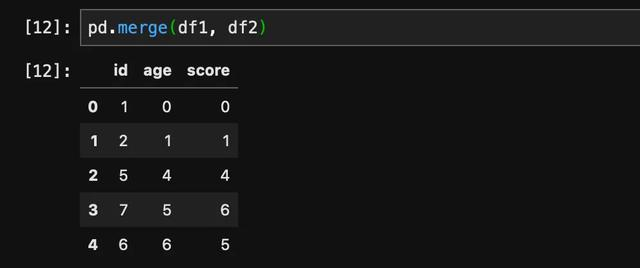```df1 = pd.DataFrame({'id': [1, 2, 3, 3, 5, 7, 6], 'age': range(7)})

df2 = pd.DataFrame({'number': [1, 2, 4, 4, 5, 6, 7], 'score': range(7)})```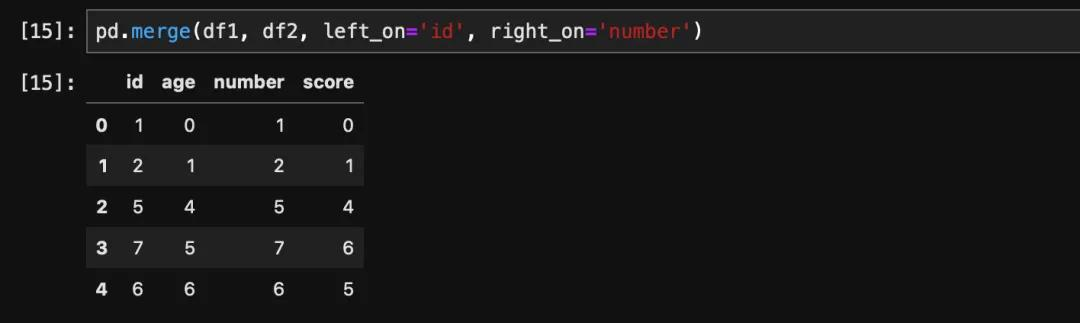join的方式选择通过how这个参数控制，比如如果我们想要左表保留，我们传入how='left'即可。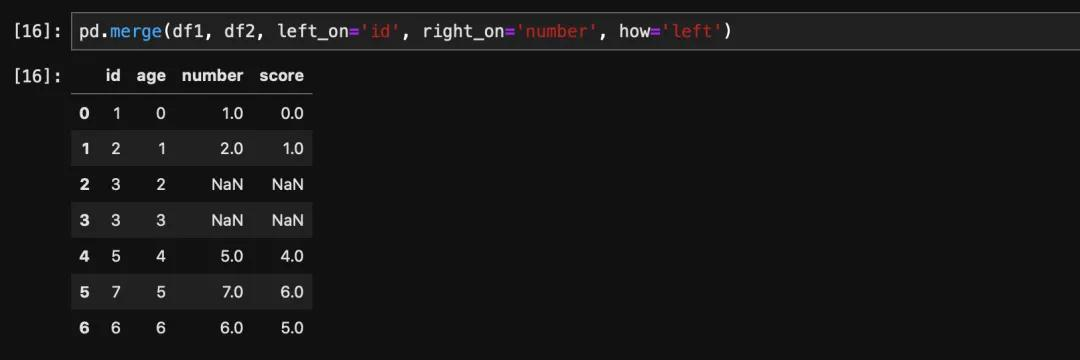```import numpy as np
arr = np.random.rand(3, 4)
```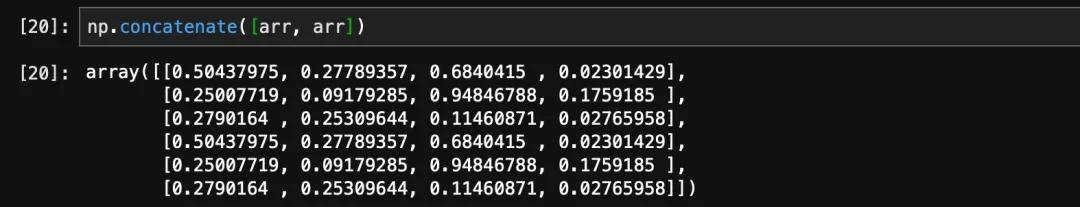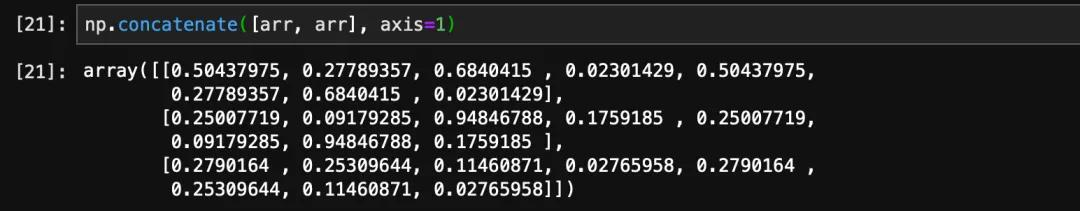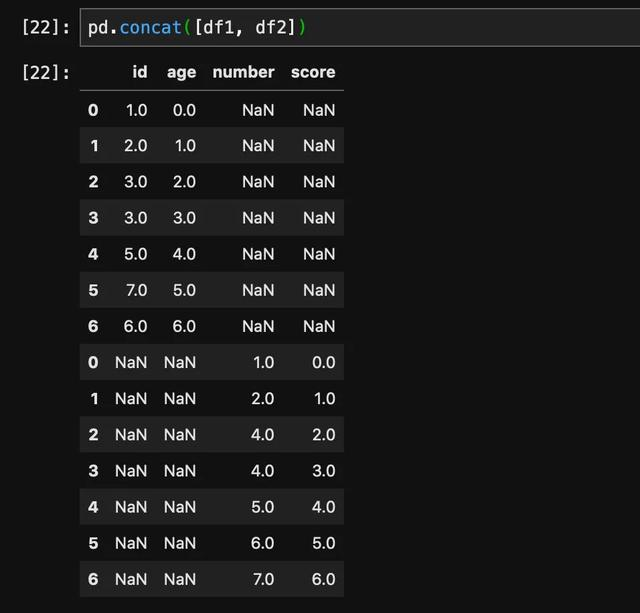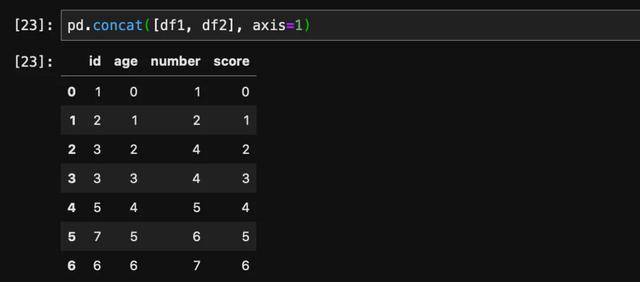OK